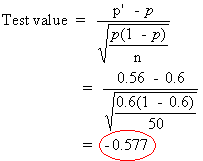### CFA Practice Question

There are 985 practice questions for this topic.

### CFA Practice Question

For the hypothesis test below, the test value is ______.A. 0.577
B. -0.04
C. -0.577
Explanation: The test value for the test of proportions is shown below.User Comment
sireklove This test of proportions is new to me... I was looking for a standard deviation to calculate the test-statistic. Where can I find this formula given in the textbook? Thanks.
wuyi SS03-C Test Statistic and significance level
uberstyle Using general formula for test statistic. However, why is variance not n*p*(1-p)?
Xocrevilo Good questions: having trawled through 100s of practice questions I have only seen this formula a few times...
leo6fin I think n is not considered because its already used in determining the value of p' = 28/50 = .56
dlukas I'm going to go ahead and settle for a 33% chance of getting this one right (on the off chance it shows up on the exam).
mjs111 At least up your chances to 50-50. Look at the numerator and denominator. The denominator will always be positive but the numerator will be either negative or positive depending on whether p' is less than or greater than p. If p' is negative then the test value should be negative.
mpapwa22 At this stage in my preparation, i think i will agree with dlukas and settle for a 33% chance. this is too much guys......lol
CJPerugini Screwed me up with that p' symbology. Usually p' means (1-p).
belikk I don't get why they have n at the bottom of the denominator.

test statistic = (population mean - hypothsized mean) / (Std Dev of sample / SQR(n) ).

Std Dev of sample = SQR(np(1-p))

Then denominator under the square root shold look like np(1-p)/n, and the n's are reduced, right?